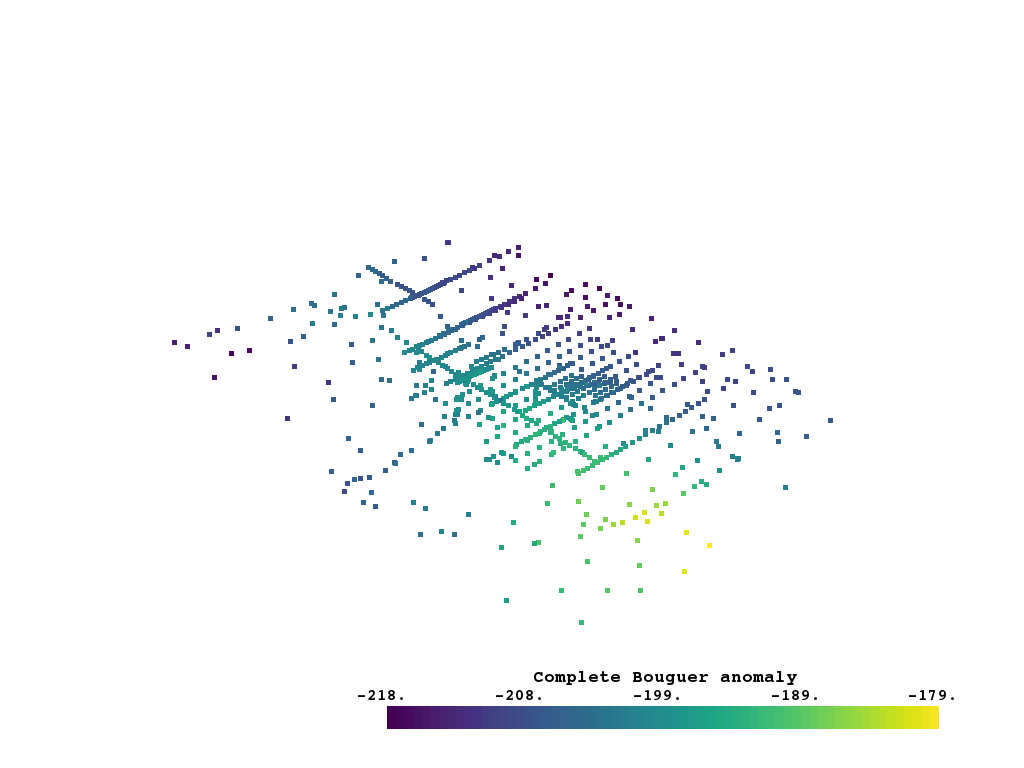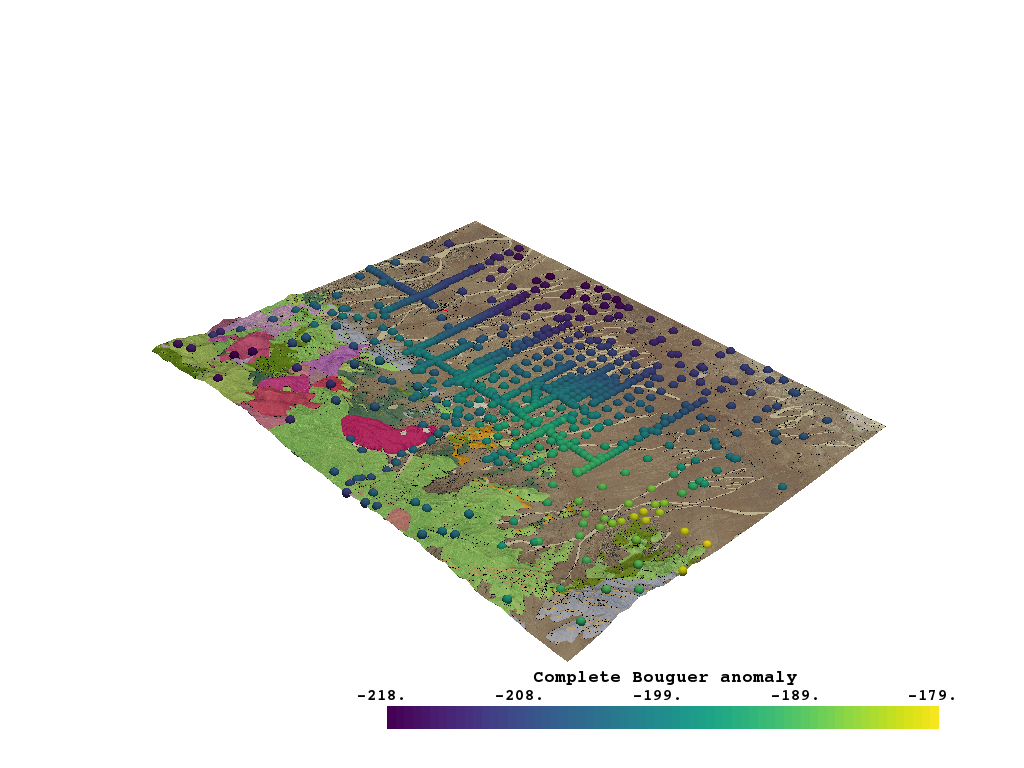# Inspect Gravity Data¶

Here we take a look at what gravity data is available for the FORGE site

```# sphinx_gallery_thumbnail_number = 2
import gdc19

import pandas as pd
import pyvista
import omfvista
```
```df = pd.read_csv(gdc19.get_gravity_path('Utah_FORGE_Gravity_Data.txt'))
print(df.keys())
```

Out:

```Index(['FID', 'FID_', 'name', 'lon', 'lat', 'easting', 'northing', 'HAE',
'NGVD29', 'obs', 'errg', 'iztc', 'oztc', 'gFA', 'gSBGA', 'gCBGA',
'Source'],
dtype='object')
```

Make a mapping between scalar names and their descriptions

```field_desc = {
"name": "the individual gravity station name.",
"lon": "station Longitude, WGS84",
"lat": "station latitude, WGS84",
"easting": "UTM zone 12 easting, NAD83",
"northing": "UTM zone 12 northing, NAD83",
"HAE": "height above ellipsoid [meter]",
"NGVD29": "vertical datum for geoid [meter]",
"obs": "observed gravity",
"errg": "gravity measurement error [mGal]",
"iztc": "inner zone terrain correction [mGal]",
"oztc": "outer zone terrain correction [mGal]",
"gFA": "free air gravity",
"gSBGA": "Bouguer horizontal slab",
"gCBGA": "Complete Bouguer anomaly",
"Source": "data source",
}
```
```ref = ['easting', 'northing', 'HAE']
points = df[ref]
for name in ref:
df.pop(name)
grav_obs = pyvista.PolyData(points.values)
grav_obs.point_arrays.update(df.to_dict('series'))

grav_obs = grav_obs.clip_box(gdc19.get_roi_bounds(), invert=False)

print(grav_obs)
```

Out:

```UnstructuredGrid (0x7ff8989cdca8)
N Cells:      786
N Points:     781
X Bounds:     3.301e+05, 3.441e+05
Y Bounds:     4.253e+06, 4.271e+06
Z Bounds:     1.488e+03, 2.706e+03
N Arrays:     14
```
```grav_obs.plot(scalars='gCBGA', stitle=field_desc['gCBGA'])
``````# Load the topography surface that was previously aggregated:
topo = surfaces['land_surface']
```
```p = pyvista.Plotter()```grav_obs.save(gdc19.get_gravity_path('grav_obs.vtk'))